#0

## Javascript SICP 3.2 - The Environment Model of Evaluation (完整解釋什麼是closure)

### Environment, frame, binding

environments 就是一個 “frame”的序列，而每個 frame 是一個 “binding”的表格(可能為空)，binding將變量與值對應起來，一個frame中的binding最多只會對應一個變量，如果變量在任何frame中都沒有定義binding，就稱為“unbound”。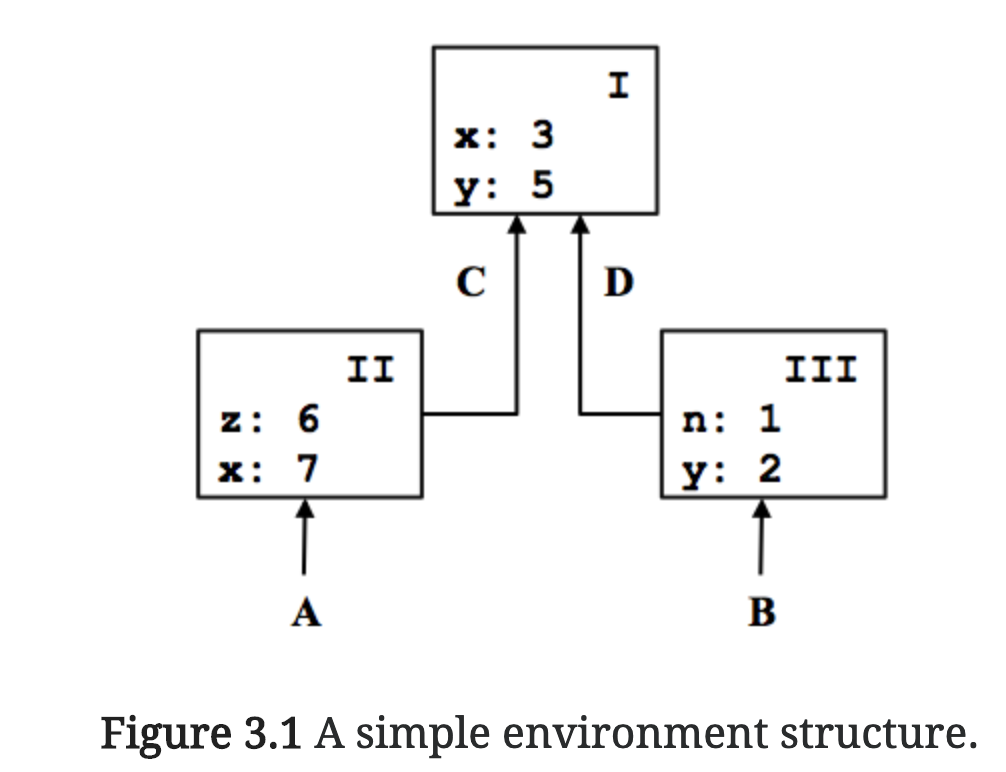Figure 3.1 有三個 frame I, II, III，觀察x,在每個frame裡面的值 I: 3, II:7, III: 3

### The Rules for Evaluation

1. Evaluate the subexpressions of combination 對組合式的子表達式求值
2. Apply the value of the function subexpression to the values of the argument subexpressions. 將子表達式function的值應用在子表達式的參數上

### Function definition

``````funciton square(x) {
return x * x;
}
``````

``````const square = x = x * x;
``````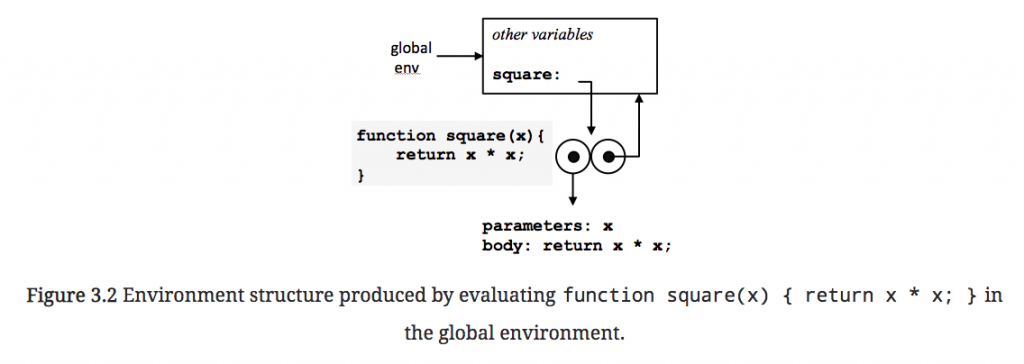### Function evaluating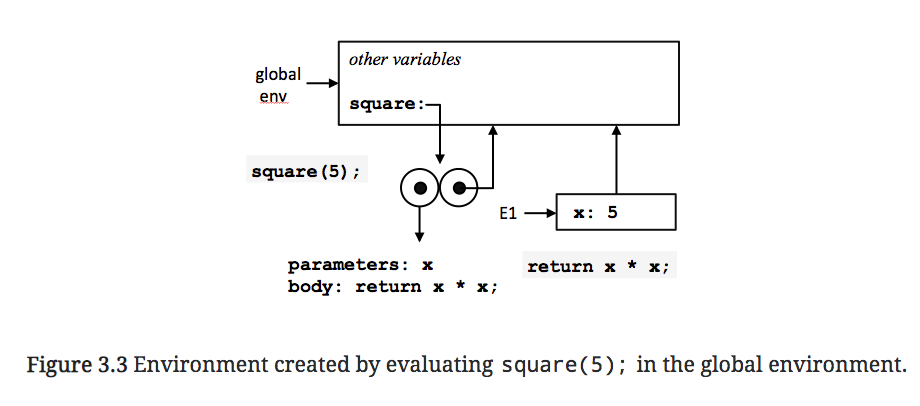1. 創造新的Environment E1，且有x變數在初始的frame中，bound to 5，pointer指向global environment.

2. square在E1對body進行求值，回傳xx，x值已綁定5，55=25。

### Applying Simple Functions

``````function square(x) {
return x * x;
}

function sum_of_squares(x, y) {
return square(x) + square(y);
}

function f(a) {
return sum_of_squares(a + 1, a * 2);
}
``````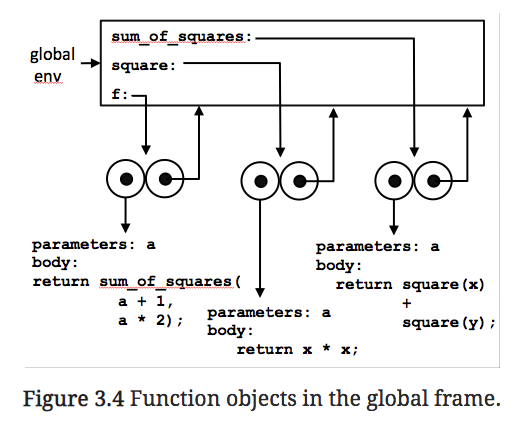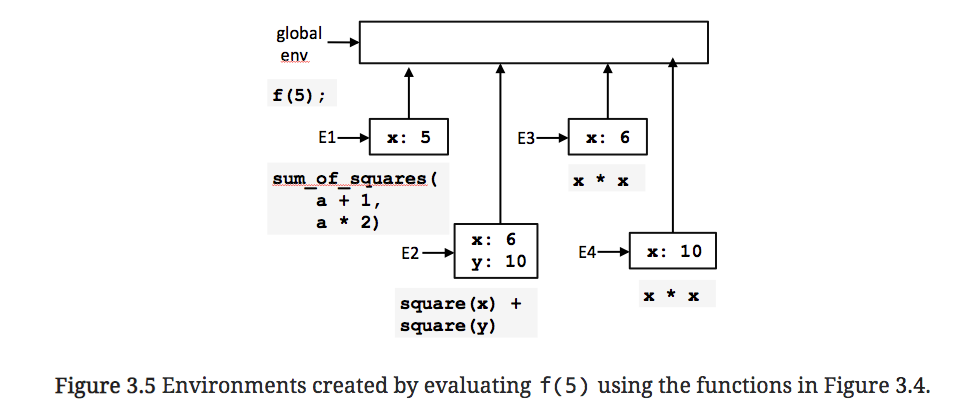### Frames as the Repository of Local State

``````function make_withdraw(balance) {
function withdraw(amount) {
if (balance >= amount) {
balance = balance - amount;
return balance;
} else {
return "Insufficient funds";
}
}
return withdraw;
}
``````

`const w1 = make_withdraw(100);`

`w1(50);`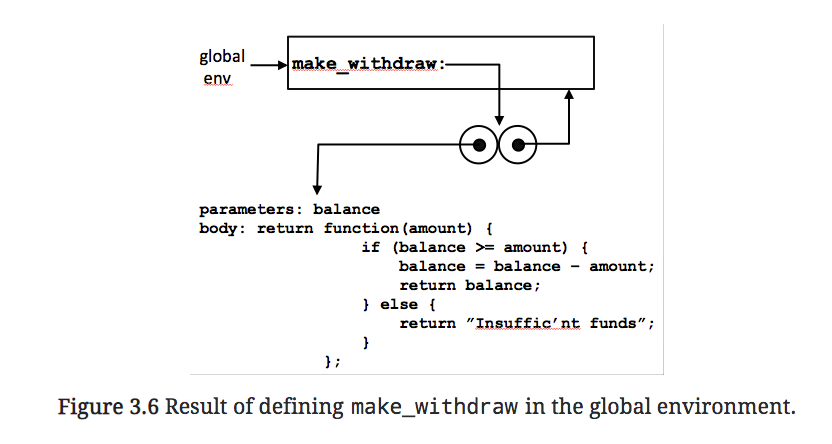`const w1 = make_withdraw(100);` 開始就非常有趣了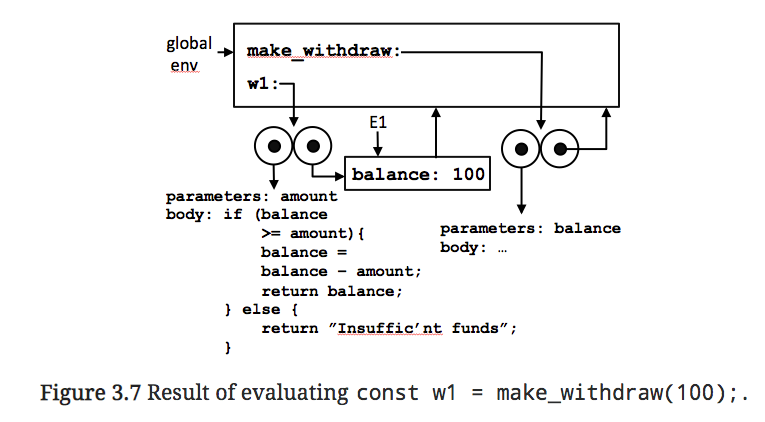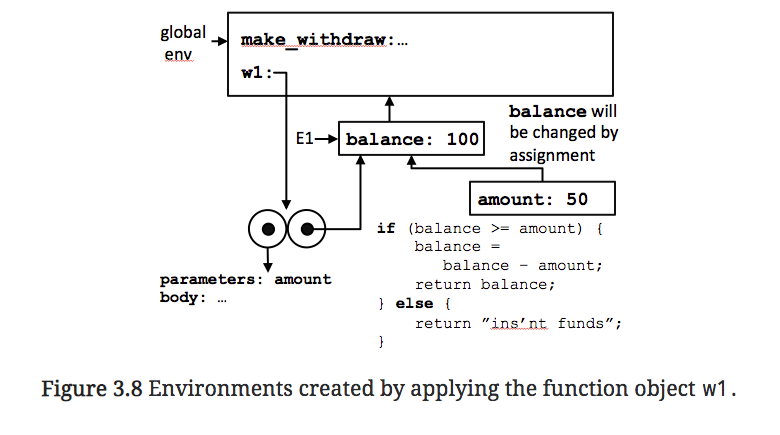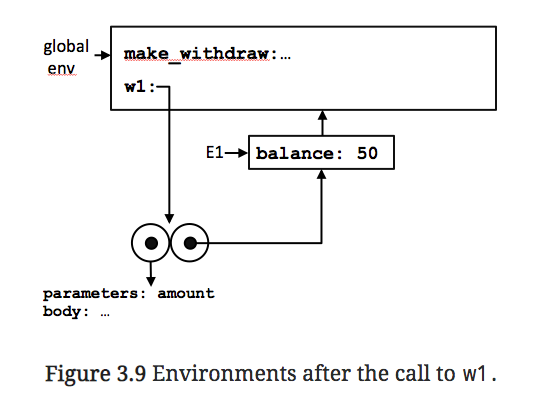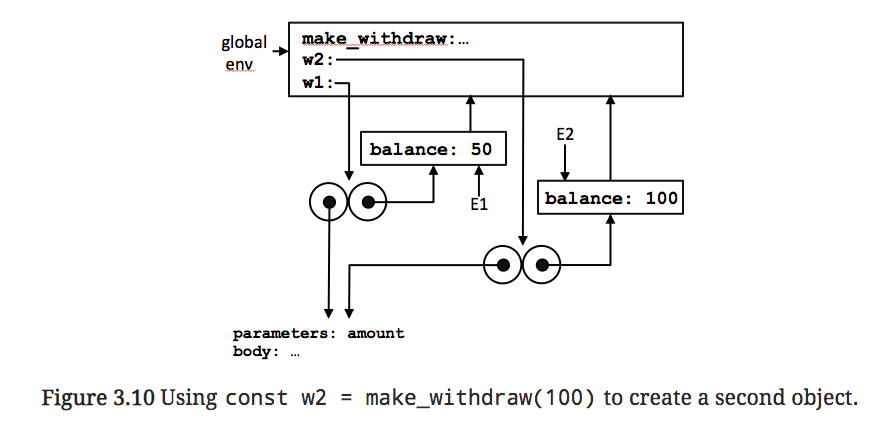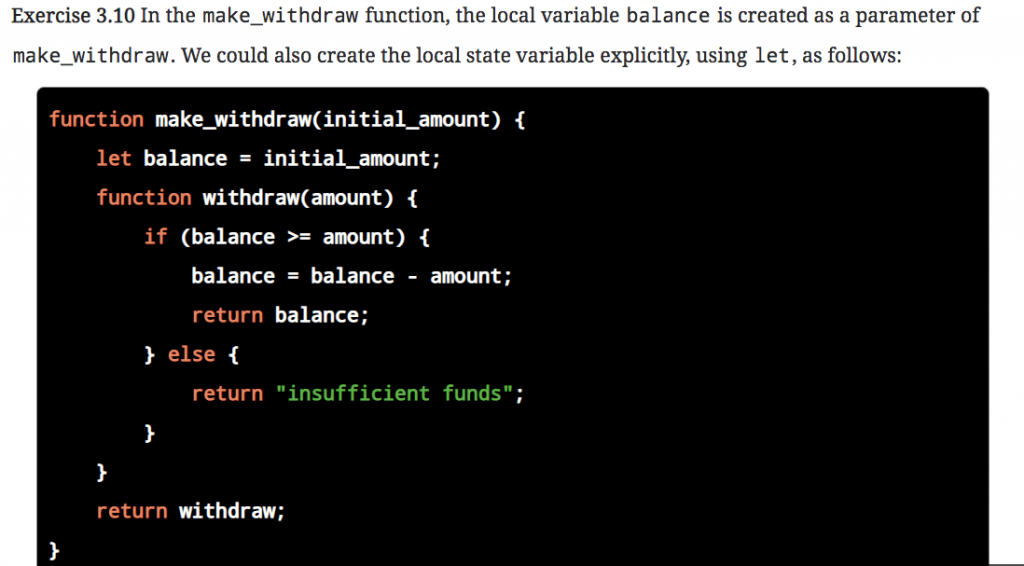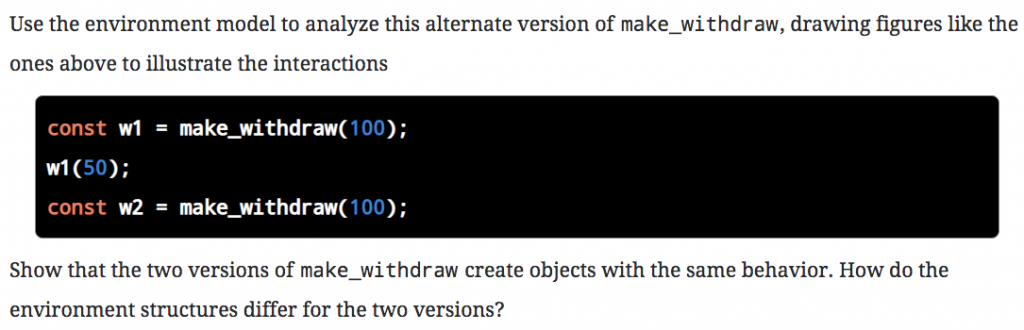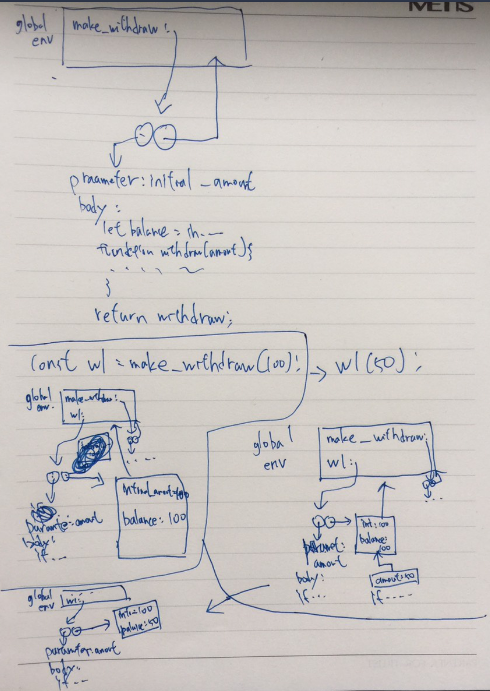### Internal Definitions

``````function abs(x) {
return x >= 0 ? x : -x;
}

function square(x) {
return x * x;
}

function average(x,y) {
return (x + y) / 2;
}

function sqrt(x) {
function good_enough(guess,x) {
return abs(square(guess) - x) < 0.001;
}
function improve(guess) {
return average(guess,x/guess);
}
function sqrt_iter(guess){
if (good_enough(guess,x)) {
return guess;
} else {
return sqrt_iter(improve(guess));
}
}
return sqrt_iter(1.0);
}

sqrt(5);
``````1. function內部的名稱不會與外部環境名稱相互干擾

2. function 內部名稱可以直接使用外部環境的，只要單純的將變數是free variables就好